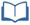# Teacher Resources

## Simply Surveying

On this page you will find lesson objectives, standards, instructional notes and UDL opportunities.

Estimated Time:  45 minutes

###Lesson Objective:

• The students will develop and collect data, emphasizing the important of simple random sampling.

Key Common Core State Standards:
S.IC.1: Understand statistics as a process for making inferences about population parameters based on a random sample from that population.

Supporting Common Core State Standards:
7.SP.1: Understand that statistics can be used to gain information about a population by examining a sample of the population; generalizations about a population from a sample are valid only if the sample is representative of that population. Understand that random sampling tends to produce representative samples and support valid inferences.

7.SP.2: Use data from a random sample to draw inferences about a population with an unknown characteristic of interest. Generate multiple samples (or simulated samples) of the same size to gauge the variation in estimates or predictions. For example, estimate the mean word length in a book by randomly sampling words from the book; predict the winner of a school election based on randomly sampled survey data. Gauge how far off the estimate or prediction might be.

Standards for Mathematical Practice Emphasized:
1. Make sense of problems and persevere in solving them.
2. Reason abstractly and quantitatively.
4. Model with mathematics.
7. Look for and make use of structure.
8. Look for and express regularity in repeated reasoning.

## Instructional Notes:

This lesson in the module focuses on the Common Core Standard S.IC.1, which states “Understand statistics as a process for making inferences about population parameters based on a random sample from that population.” The lesson begins with the students reviewing what statistical procedures produce a simple random sample. From this, the students gather a random sample from chosen populations. In the subsequent lesson, the students will continue with Standard S.IC.1 and make inferences and draw conclusions about the samples they collect.

Take time to read pp. 8 – 12 of the Progressions for the Common Core State Standards in Mathematics. This document describes the progression of knowledge and understanding the students should experience as they work with Standard S.IC.1. According to this document, “Students now move beyond analyzing data to making sound statistical decisions based on probability models” (p. 8). The authors of this document identify the reasoning process as follows:

• Develop a statistical question in the form of a hypothesis (supposition) about a population parameter.
• Choose a probability model for collecting data relevant to that parameter.
• Collect data.
• Compare the results seen in the data with what is expected under the hypothesis.
This first lesson focuses on the development and collection of data, emphasizing the importance of simple random sampling. In the second lesson, Predicting the Future, the students will begin to make predictions based off of the sample data collected. They will make conclusions and draw inferences about the samples, comparing the results to the hypotheses they form.

> Go to Simply Surveying lesson

## Sample Responses

For sample responses to the Algebra II Journal questions, visit the Algebra II Journal in the Teacher Resources.

## Simply Surveying - Page 2

Students are familiar with random samples and sampling populations from their work in Common Core Mathematics Grade 7. This introductory activity is intended to remind the students of what makes a sample a simple random sample.

> Go to lesson, page 2

## Simply Surveying - Page 7

The following activity can be used as a formative assessment for this lesson. The purpose of the activity is to show students why samples need to be simple random samples. In the activity, students will first obtain a sample that is biased because the sample is selected by choice. Students then obtain a sample using a random number generator. (Note, students will need a graphing calculator for this.) After comparing the distribution of the samples, students should see that a random sample better represents a population. As an extension to this activity, combine the class data for the random samples. This will re-emphasize the fact that large samples as well as random sampling better represents the population.

> Go to lesson, page 7

## UDL Opportunities:

Principle 1: Provide Multiple Means of Representation
Checkpoint 1.1 Offer ways of customizing the display of information
Checkpoint 1.3 Offer alternatives for visual information
Checkpoint 2.1 Clarify vocabulary and symbols
Checkpoint 2.3 Support decoding text, mathematical notation, and symbols
Checkpoint 2.5 Illustrate through multiple media
Checkpoint 3.1 Activate or supply background knowledge
Checkpoint 3.2 Highlight patterns, critical features, big ideas, and relationships
Checkpoint 3.3 Guide information processing, visualization, and manipulation
Checkpoint 3.4 Maximize transfer and generalization

Principle 2: Provide Multiple Means of Action and Expression
Checkpoint 4.1 Vary the methods for response and navigation
Checkpoint 5.1 Use multiple media for communication
Checkpoint 6.2 Support planning and strategy development
Checkpoint 6.3 Facilitate managing information and resources

Principle 3: Provide Multiple Means of Engagement
Checkpoint 7.2 Optimize relevance, value, and authenticity
Checkpoint 7.3 Minimize threats and distractions
Checkpoint 8.1 Heighten salience of goals and objectives
Checkpoint 8.4 Increase mastery-oriented feedback
Checkpoint 9.3 Develop self-assessment and reflection